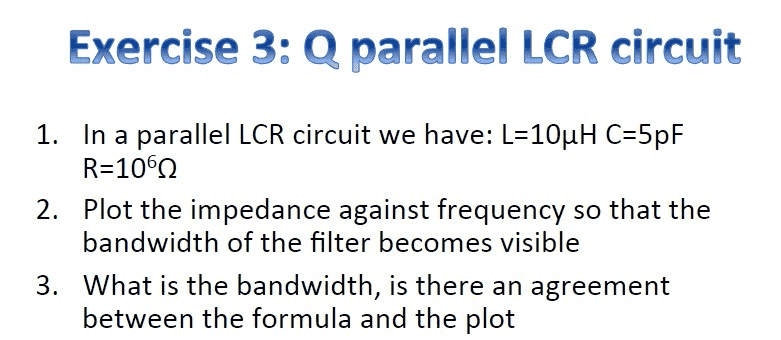# Finding the bandwidth of a parallel RLC circuit (+MATLAB)

• Engineering
ItsTheSebbe
Homework StatementThe attempt at a solution
Constructing the total impedance of the circuit as follows,
$$\frac{1}{Z_T}=\frac{1}{Z_R}+\frac{1}{Z_C}+\frac{1}{Z_L}$$
where $Z_R=R$, $Z_C=-j\frac{1}{\omega C}$ and $Z_L=j\omega L$.
$$\frac{1}{Z_T}=\frac{1}{R}+j\omega C+\frac{1}{j\omega L}$$
solving for $Z_T$ gives us,
$$Z_T=\frac{Rj\omega L}{j\omega L-RCL\omega^2+R}$$
where $\omega=2\pi f$
$$Z_T=\frac{2\pi fRLj }{2\pi fLj-RCL(2\pi f)^2+R}$$
Plotting this function over the frequencies, whilst $L=10\ \mu H$, $C=5\ pF$ and \$R=10^6\ \Omega

From here, I created the following MATLAB code to find:
- The magnitude of the impedance (Z_Mag) for a range of frequencies.
- Finding the maximum of Z_mag and it's corresponding frequency.
- finding the -3db points and the frequencies that correspond to these points.
- taking the difference of said frequencies in order to calculate the bandwidth.
Code:
clear all
close all

N=100000; %number of frequency samples
L=10*10^(-6); %inductance
C=5*10^(-12); %capacitance
R=10^6; %resistance
f=linspace(1,10^8,N); %frequency of 1 Hz to 100 MHz
df=f(2)-f(1);

Z_T=(2*pi*f*R*L*1i)./(2*pi*f*L*1i-R*C*L*(2*pi*f).^2+R); %impedance
Z_mag=abs(Z_T); %magnitude of the complex impedance

plot(f,Z_mag); %plotting the frequency against the total impedance
title('Total impedance per frequency')
xlabel('frequency')
ylabel('total impedance')

[max_Z, max_index]=max(Z_mag); %maximum value of impedance
threedb=max_Z*sqrt(2)/2; %the 3db point

[Z_db,index_db] = min(abs(Z_mag-threedb)); %closest value and index to the 3db point
f1=index_db*df; %the first frequency at the 3db point
%the second frequency at the 3db point (first frequency mirrored around the
%frequency at max magnitude
f2=(max_index+(max_index-index_db))*df;

BW=abs(f2-f1); %the bandwidth

Now when I vary the N (number of frequency samples), the bandwidth I find seems to change quite drastically. Ideally, I would expect the bandwidth to asymptotically approach some value with a greater accuracy for a greater N.

I have been trying to found out what I did wrong for some time now, does anyone know where I went wrong? Thanks!

#### Attachments

Last edited:

•